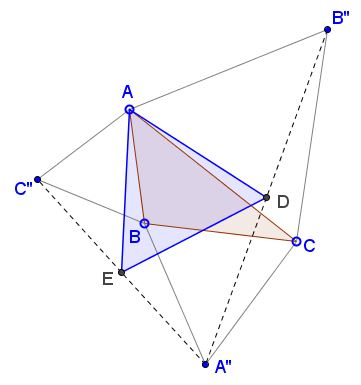# Another Equilateral Triangle in Napoleon's Configuration

## What is this about? A Mathematical Droodle

ExplanationThe applet attempts to suggest a problem communicated to me by Marco Antôumflex;nio Manetta at the CutTheKnotMath facebook page:

Form equilateral triangles $ABC'',\;$ $AB''C,\;$ $A''BC\;$ on the sides of $\Delta ABC\;$ and define $D = \frac{1}{2}(A''+B'')\;$ and $E=\frac{1}{2}(A''+C'').$Prove that $\Delta ADE\;$ is equilateral.

### Proof 1

We identify the points in the plan with complex numbers. Let $d\;$ be a counterclockwise rotation around origin through $60^{\circ}$ and place the origin at the center of $\Delta ABC.\;$ This implies $A+B+C=0.$

Then the construction is equivalent to the following definitions:

\displaystyle\begin{align} A''&=C+d(B-C),\\ B''&=A+d(C-A),\\ C''&=B+d(A-B),\\ D&=\frac{A''+B''}{2}=\frac{A+C}{2}+\frac{d}{2}(B-A)=-\frac{B}{2}+\frac{d}{2}(B-A),\\ E&=\frac{A''+C''}{2}=\frac{B+C}{2}+\frac{d}{2}(A-C)=-\frac{A}{2}+\frac{d}{2}(A-C). \end{align}

What needs to be proved now is

(*)

$d(E-A)=D-A.$

This is reasonably straightforward. We only need to take into account that $d^2-d+1=0.$

\displaystyle\begin{align} d(E-A) &= d(-\frac{A}{2}+\frac{d}{2}(A-C)-A)\\ &=-\frac{3d}{2}A+\frac{d^2}{2}(A-C)\\ &=-\frac{3d}{2}A+\frac{d-1}{2}(A-C)\\ &=-dA-\frac{d}{2}C-\frac{1}{2}(A-C), \end{align}

whereas

\displaystyle\begin{align} D-A &= -\frac{B}{2}+\frac{d}{2}(B-A)-A\\ &=-\frac{C-A}{2}+\frac{d}{2}(B-A). \end{align}

Thus we need only to compare $\displaystyle -dA-\frac{d}{2}C\;$ and $\displaystyle \frac{d}{2}(B-A),\;$ or $-2A-C$ and $B-A.\;$ The two quantities are equal: $2A+C+B-A=A+B+C=0.\;$

### Proof 2

The spectral proof is by Grégoire Nicollier.

Define the three triangle of the Fourier basis: $F_0=(1,1,1),\;$ $F_1=(1,w,w^2)\;$ with $\displaystyle w=e^{2i\frac{\pi}{3}},\;$ $F_2=(1,w^2,w).\;$. The transformation maps $F_0\;$ to $F_0,\;$ $F_1\;$ to $F_2,\;$ and $F_2\;$ to $\frac{1}{2}(F_0+F_2),\;$ leaving the vertex $1\;$ fixed. Thus $\Delta ABC = aF_0 + bF_1 + cF_2\;$ is mapped to $\displaystyle (a+\frac{c}{2})F_0 + (b+\frac{c}{2})F_2,\;$ which is equilateral.### Napoleon's Theorem